## Monday, December 17, 2018

### A phylogenetic Christmas tree in R (adapted from Gustavo Ballen)

Today, a colleague Gustavo Ballen shared an cleverly decorated phylogenetic Christmas tree that he created using the phylogenetic visualization software, FigTree.

I hope that he doesn't mind that I'm sharing a script to recreate his plot in R:

``````library(phytools)
library(plotrix)
library(phangorn)
tree<-stree(20,"left")
tree\$tip.label<-paste("taxon_",1:20,sep="")
for(i in 1:tree\$Nnode) if(i%%2==1) tree<-rotate(tree,Ntip(tree)+i)
tree\$edge.length<-c(0,rep(1,nrow(tree\$edge)-1))
tree<-di2multi(tree)
plot.new()
par(mar=rep(0.1,4))
plot.window(xlim=c(0.5,20.5),ylim=c(-1,20))
lines(c(10.5,10.5),c(-1,-0.2),lwd=15,col="brown",lend=2)
plotTree(tree,direction="upwards",ftype="i",fsize=0.7,offset=0.5,
plotTree(tree,direction="upwards",ftype="i",fsize=0.7,offset=0.5,
lastPP<-get("last_plot.phylo",envir=.PlotPhyloEnv)
for(i in 1:tree\$Nnode){
node<-i+Ntip(tree)
daughters<-Children(tree,node)
daughters<-daughters[c(1,length(daughters))]
xx<-lastPP\$xx[daughters]
yy<-rep(lastPP\$yy[node],2)
cols<-if(i%in%c(2,5,6,9,10,13,14,17,18)) c("red","yellow") else
c("yellow","red")
draw.circle(xx-0.1,yy-0.25,0.25,col=cols)
draw.circle(xx+0.1,yy-0.25,0.25,col=cols)
length.out<-floor(diff(xx)/4)+2
if(length.out>2){
xx<-seq(xx,xx,length.out=length.out)
if(length(xx)==3) xx<-if(xx>mean(range(lastPP\$xx)))
xx+0.25*(xx-xx) else xx-0.25*(xx-xx)
xx<-xx[2:(length(xx)-1)]
cols<-if(i%in%c(2,5,6,9,10,13,14,17,18)) rep(c("yellow","red"),
ceiling(length(xx)/2)) else rep(c("red","yellow"),
ceiling(length(xx)/2))
for(j in 1:length(xx)) draw.circle(xx[j],yy-0.25,0.25,
col=cols[j])
}
}
``````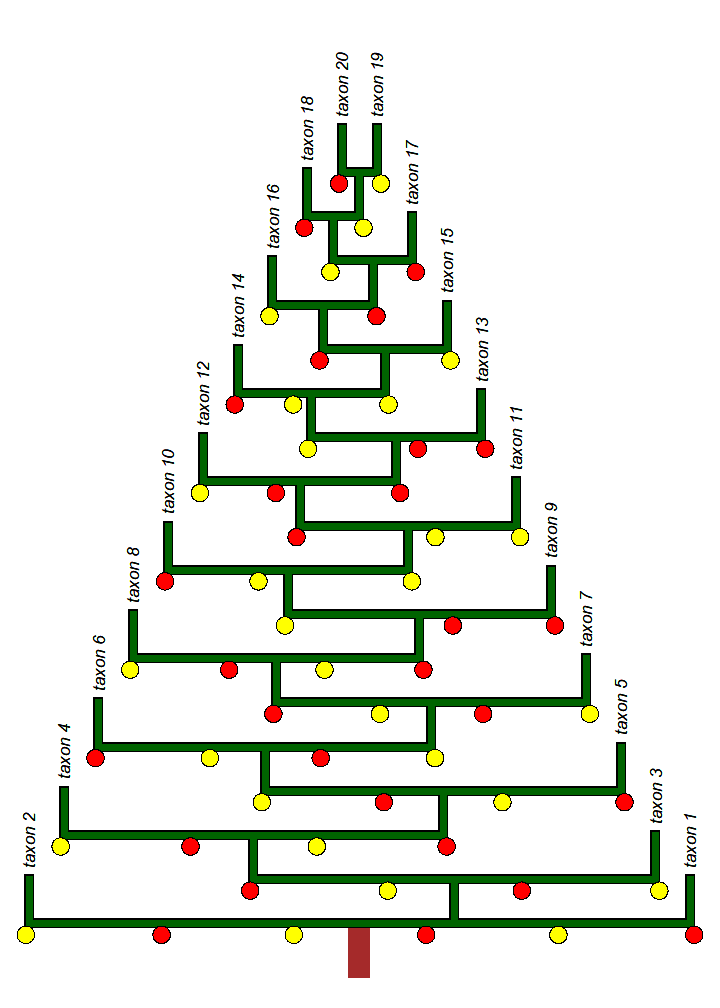Happy holidays!

## Friday, November 30, 2018

### Plotting confidence intervals on internal nodes in a traitgram

The popular visualization called a traitgram (e.g., Evans et al. 2009) is a a projection of the tree into a space that is defined by time since the root on one axis and the observed or reconstructed value of a phenotypic trait on the other. For example, here is a traitgram created using simulated data:

``````library(phytools)
tree
``````
``````##
## Phylogenetic tree with 16 tips and 15 internal nodes.
##
## Tip labels:
##  A, B, C, D, E, F, ...
##
## Rooted; includes branch lengths.
``````
``````x
``````
``````##          A          B          C          D          E          F
##  2.0275876  3.3977458  2.2337622 -3.7190991 -6.8014316 -6.6150166
##          G          H          I          J          K          L
## -8.6625154 -5.0548866 -7.9049386 -7.2639810 -1.8821460 -0.8618768
##          M          N          O          P
## -3.0035373 -6.0933141 -4.9157566  2.6977773
``````
``````phenogram(tree,x,color="darkgrey",lwd=3,ftype="i")
``````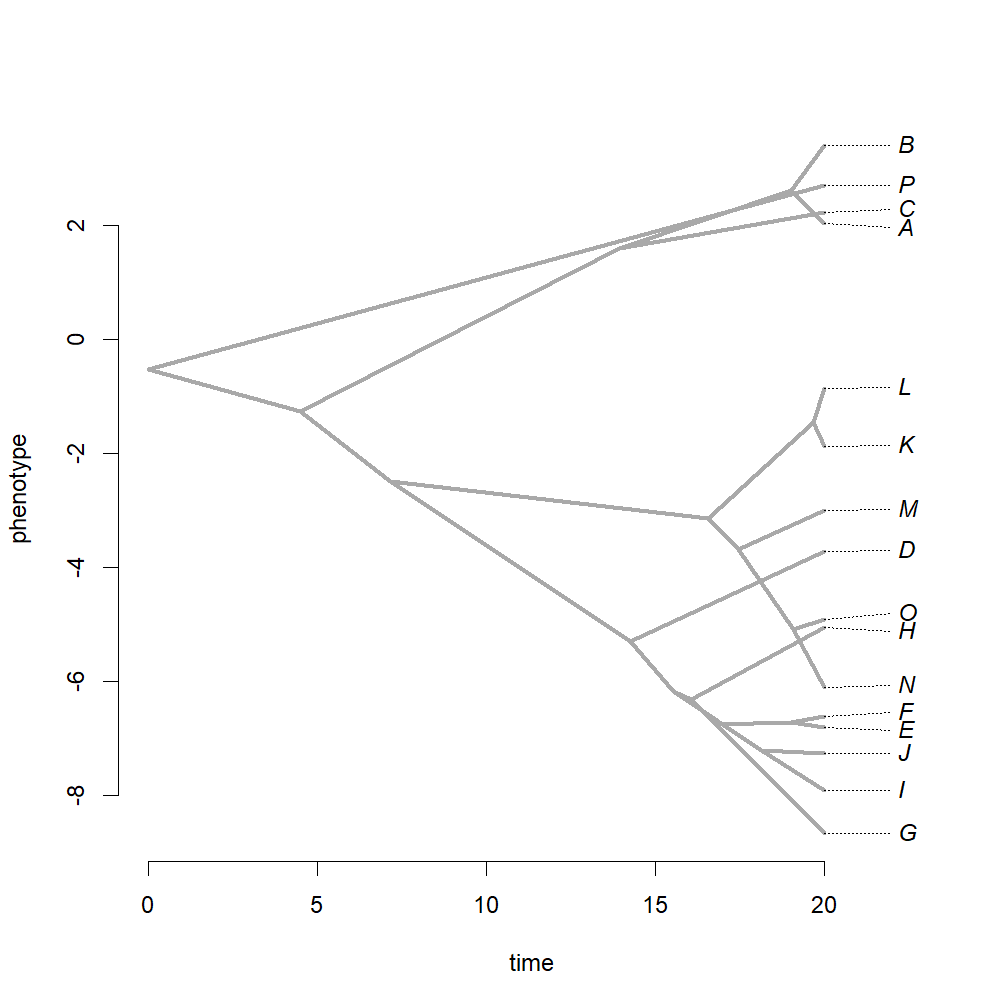In my opinion, it is completely fine to treat the vertical positions of the internal nodes of this tree merely as convenient positions from which to project the tree into this space; however, their values are (normally) the MLEs of ancestral states. Consequently, I earlier developed a method to overlay the inherent uncertainty about these values by way of a color transparency gradient. For example:

``````fancyTree(tree,type="phenogram95",x=x)
``````
``````## Computing density traitgram...
``````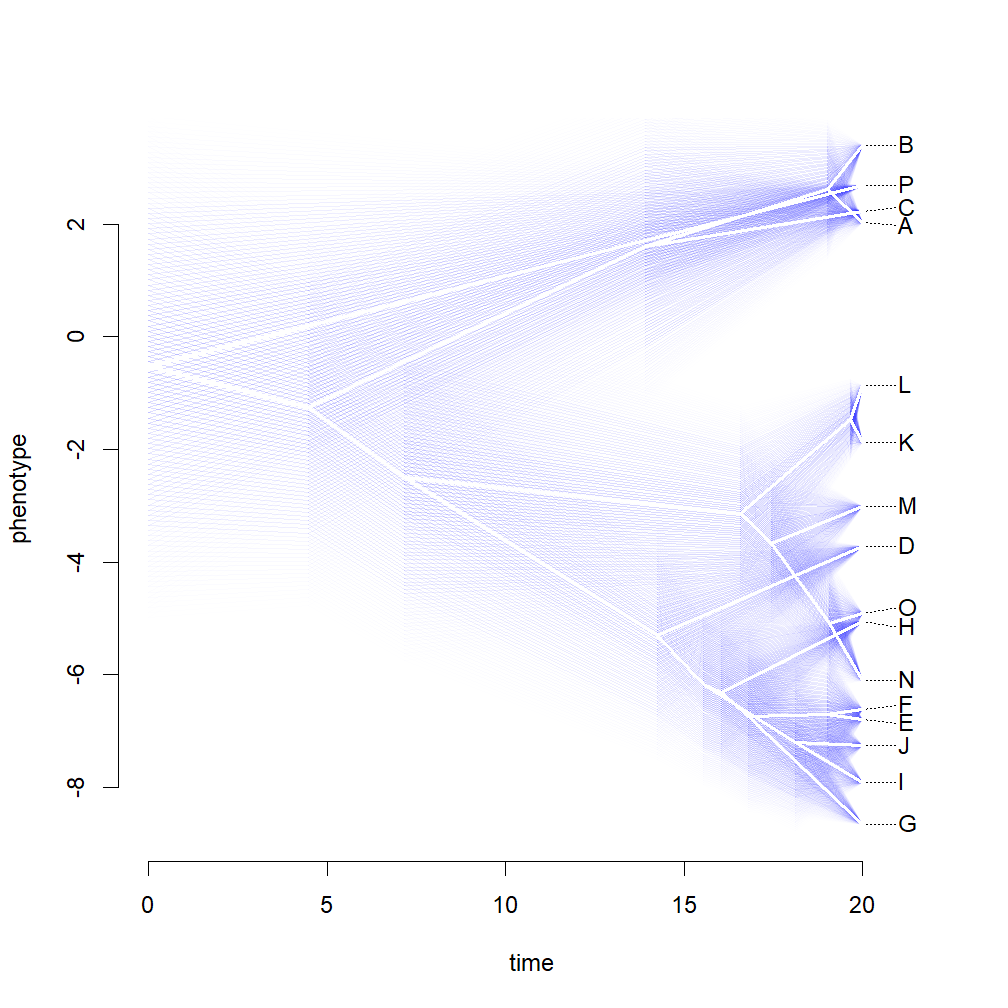Alternatively, it occurred to me that it might be equally (if not more) useful to merely overlay the 95% CIs around each internal node as a vertical line. This has never been automated, but can be done easily as follows:

``````fit<-fastAnc(tree,x,CI=TRUE)
phenogram(tree,x,ylim=range(c(x,fit\$CI95)),ftype="i")
h<-sapply(Ntip(tree)+1:tree\$Nnode,nodeheight,tree=tree)
for(i in 1:tree\$Nnode)
lines(rep(h[i],2),fit\$CI95[i,],lwd=3,
col=make.transparent("blue",0.15))
points(h,fit\$ace,cex=1.5,pch=21,bg="grey")
``````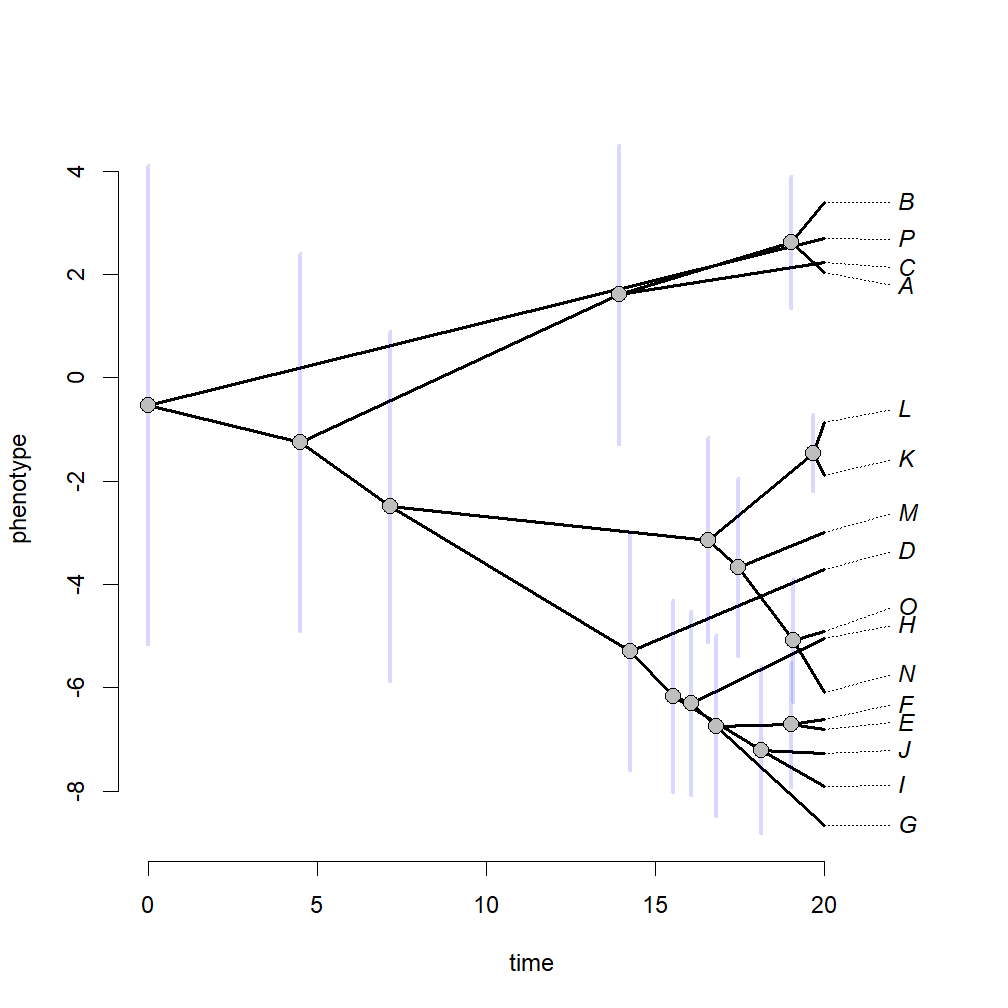Of course, since these data come from simulation we can actually know the true ancestral state values. Let's overlay them now so we can see how frequently they fall on the 95% CIs:

``````phenogram(tree,x,ylim=range(c(x,fit\$CI95)),ftype="i")
h<-sapply(Ntip(tree)+1:tree\$Nnode,nodeheight,tree=tree)
for(i in 1:tree\$Nnode)
lines(rep(h[i],2),fit\$CI95[i,],lwd=3,
col=make.transparent("grey",0.5))
points(h,fit\$ace,cex=1.5,pch=21,bg="grey")
points(h,a,cex=1.5,pch=21,bg=make.transparent("blue",0.15),col="grey")
legend(x="bottomleft",c("estimated state","true value"),pt.cex=1.5,
pt.bg=c("grey",make.transparent("blue",0.15)),pch=21)
``````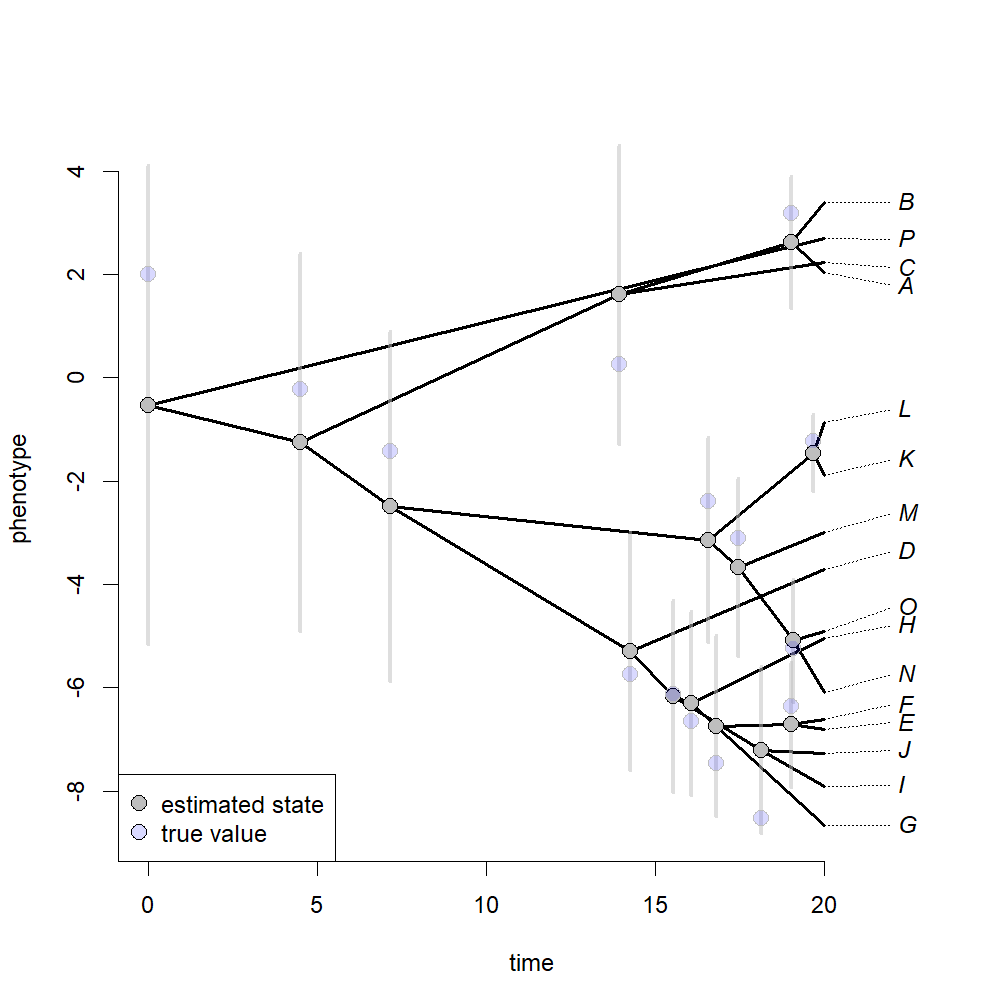Finally, let's take a look at how ancestral state estimation improves if we include a known value - say the state at the root which I happen to know is 2:

``````a
``````
``````## 17
##  2
``````
``````fit<-fastAnc(tree,x,anc.states=a,CI=TRUE)
phenogram(tree,c(x,fit\$ace),ylim=range(c(x,fit\$CI95)),ftype="i")
h<-sapply(Ntip(tree)+1:tree\$Nnode,nodeheight,tree=tree)
for(i in 1:tree\$Nnode)
lines(rep(h[i],2),fit\$CI95[i,],lwd=3,
col=make.transparent("grey",0.5))
points(h,fit\$ace,cex=1.5,pch=21,bg="grey")
points(h,a,cex=1.5,pch=21,bg=make.transparent("blue",0.15),col="grey")
``````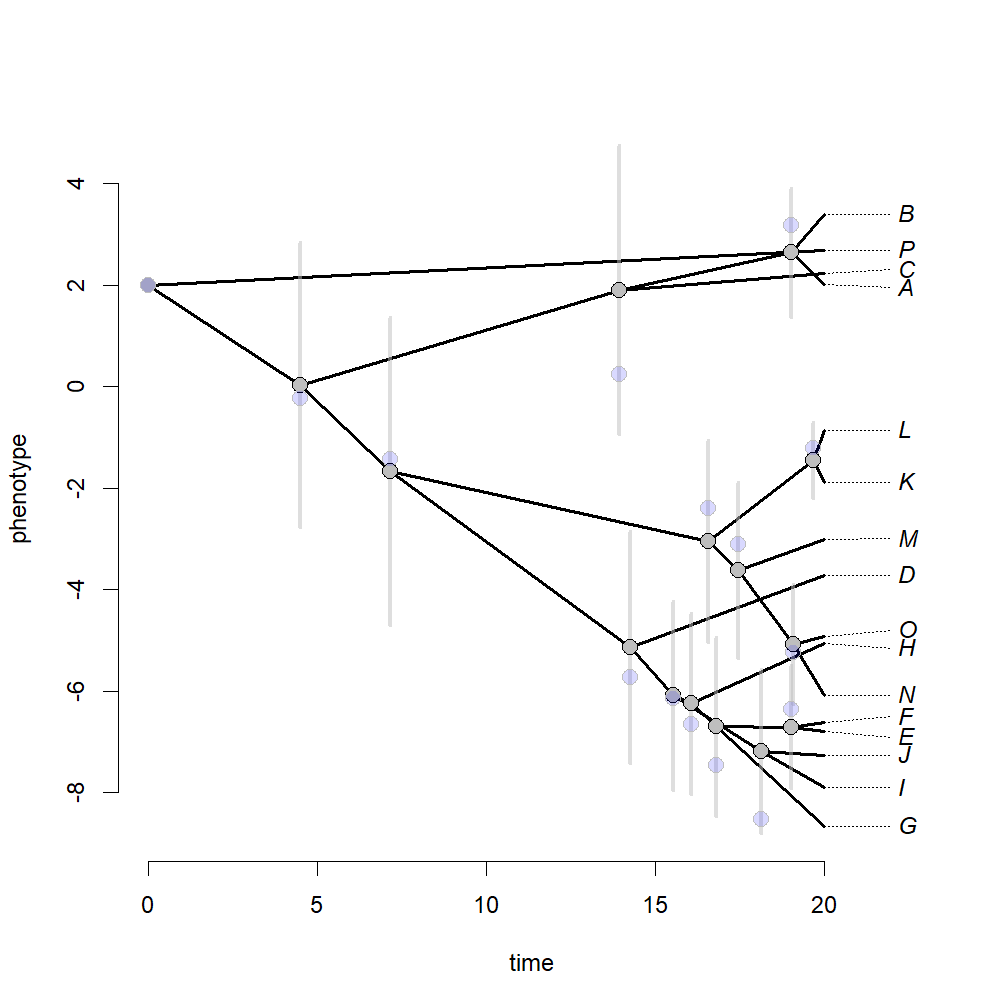Mostly deep nodes get a lot better, in this case, whereas recent nodes are relatively unaffected.

The data & tree were simulated as follows:

``````set.seed(9)
tree<-pbtree(n=16,tip.label=LETTERS[1:16],scale=20)
x<-fastBM(tree,a=2,internal=TRUE)
a<-x[Ntip(tree)+1:tree\$Nnode]
x<-x[tree\$tip.label]
``````